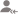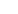true# Graphical Representation of Data Updates

Post a Lesson

All

All

Lessons

Discussion

Lesson Posted on 24/07/2018 IT Courses/R Programming IT Courses/Advanced Statistics IT Courses/Data Analysis +2 IT Courses/Data Science Graphical Representation of Data lessGoutam Dutta

I completed my education from the most reputed universities like IIEST, Shibpur and Jadavpur University....

I frequently asked the below question from my students: 'Do I You need Statistics to learn R Programming?' The answer is, NO. If you want to learn R programming only, Stat is not required. You can be an expert R programmer without knowing anything of Stat because R is a language where lot of APIs are... read more

I frequently asked the below question from my students:

'Do I You need Statistics to learn R Programming?'

The answer is, NO. If you want to learn R programming only, Stat is not required. You can be an expert R programmer without knowing anything of Stat because R is a language where lot of APIs are provided for Statistical calculations and Visualization.

But, if you want to get the flavour of Big Data Analysis and Data Visualization, basic Stat knowledge will help. If you want to be a Data Scientist, Stat is very much required. In this case, I will suggest you to take a Stat course before starting R Programming learning or in parallel.

Dislike Bookmark

Answered on 19/11/2016 CBSE/Class 10/Mathematics Tuition/Class IX-X Tuition Graphical Representation of DataManan Kenia

Internal Auditor, Financial Analyst

Natural numbers = 1,2,3,4, & so on... Mean = (1+2+3+4+5) / 5 = 15/5 = 3
Dislike Bookmark

Answered on 03/12/2016 CBSE/Class 10/Mathematics Tuition/Class IX-X Tuition Graphical Representation of Data

Define frequency polygon?Neelabh Tiwari

A Good Teacher

Frequency polygon is a graphical device which is used for understanding the shapes of distributions. It also give the idea about the data distribution.
Dislike Bookmark

Answered on 24/11/2016 CBSE/Class 10/Mathematics Tuition/Class IX-X Tuition Graphical Representation of Data

Find the class mark of the class 130 - 150?More Than 15 Years Experiece

20.
Dislike Bookmark

Answered on 01/12/2016 CBSE/Class 10/Mathematics Tuition/Class IX-X Tuition Graphical Representation of Data

Bar diagram is a ______ dimensional diagram.Kusuma S.

Both 2 and 3 dimensional diagram.
Dislike Bookmark

Answered on 22/11/2016 CBSE/Class 10/Mathematics Tuition/Class IX-X Tuition Graphical Representation of Data

What is Assume Mean Method?Chandan Singh

Tutor

In statistics the assumed mean is a method for calculating the arithmetic mean and standard deviation of a data set. It simplifies calculating accurate values by hand. Its interest today is chiefly historical but it can be used to quickly estimate these statistics.
Dislike Bookmark

Answered on 02/12/2016 CBSE/Class 10/Mathematics Tuition/Class IX-X Tuition Graphical Representation of Data

Find the mean daily expenditure on food by a suitable method of a given data: Daily expenditure 100... read more
Find the mean daily expenditure on food by a suitable method of a given data: Daily expenditure 100 - 150 150 - 200 200 - 250 250 - 300 300 - 350 No. of households 4 5 12 2 2 read lessAbhijeet Kumar Jha

Math magician

211
Dislike Bookmark

Answered on 02/12/2016 CBSE/Class 10/Mathematics Tuition/Class IX-X Tuition Graphical Representation of Data

Define modal class?Abhijeet Kumar Jha

Math magician

Modal class are those class in which frequency occurs most.
Dislike Bookmark

Answered on 24/11/2016 CBSE/Class 10/Mathematics Tuition/Class IX-X Tuition Graphical Representation of Data

What is the formula for finding median of odd observation?Mamta Mund

Maths Tutor

Arrange in ascending or descending order for the data. Find (n+1) /2 th observation where, n is the number of observation.
Dislike Bookmark

Answered on 24/11/2016 CBSE/Class 10/Mathematics Tuition/Class IX-X Tuition Graphical Representation of Data

What is the difference between mean, median, mode?Mamta Mund

Maths Tutor

Mean is the Average. Median is the middle most value either in ascending or descending order. Mode is the value of highest frequency.
Dislike Bookmark

UrbanPro.com helps you to connect with the best in India. Post Your Requirement today and get connected.

Overview

Questions 21

Total Shares38 Followers

## Top Contributors

Connect with Expert Tutors & Institutes for Graphical Representation of Datax